## Tuesday, January 01, 2008

### Proof for Cotes' Formulas

In today's blog, I will show how De Moivre's Formula can be used to establish Cotes' Formulas.

Lemma 1:

Let μn be the set of all nth roots of unity

Then:Proof:

(1) We know that xn - 1 has n root from the Fundamental Theorem of Algebra.

(2) We also note that for all ζ, we have )n = 1

(3) Based on #2, the Fundamental Theorem of Algebra gives us:

xn - 1 = (x - ζ1)*(x - ζ2)*(x - ζ3)*...*(x - ζn)

(4) Step #3 can then be restated as:QED

Corollary 1.1:Proof:

This follows from applying De Moivre's proof [see Corollary 1.1, here] for the existence of the roots of complex numbers with the result in Lemma 1 above.

QED

Corollary 1.2: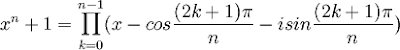Proof:

This follows from applying De Moivre's proof [see Corollary 1.2, here] for the existence of the roots of complex numbers with the result in Lemma 1 above.

QED

Theorem 2:

If n is odd: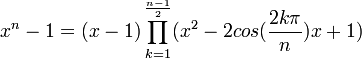If n is even:Proof:

(1) From Corollary 1.1 above, we have:(2) Now, we can remove the k=0 case to get the following:(3) Now, it is also possible to pair up roots. That is, let l = n - k.

(4) Then we have:

cos(2[(n-k)π]/n) =
=cos([2nπ - 2kπ]/n) =
=cos([2nπ/n] - [2kπ/n]) =
=cos(2π - 2kπ/n) =
cos(-2kπ/n) = cos(2kπ/n) [See Property 9, here]

sin(2[(n-k)π]/n) =
=sin([2nπ - 2kπ]/n) =
=sin([2nπ/n] - [2kπ/n]) =
=sin(2π - 2kπ/n) =
=sin(-2kπ/n) = -sin(2kπ/n) [See Property 4, here]

(5) So, pairing the roots of unity k with its n-k pair gives us: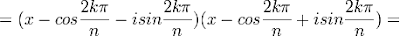(6) So, if n is odd, we have:(7) If n is even, then at k=n/2 we have:

cos([2(n/2)π]/n) = cos(nπ/n) = cos(π) = -1 [See Property 6, here]

sin([2(n/2)π]/n) = sin(nπ/n) = sin(π) = 0 [See Property 1, here]

So:

[x - cos([2(n/2)π];/n) - isin([2(n/2)π]/n)] =

= x - (-1) - i*0 = x + 1

(8) So, if n is even, we have:since we can see that n/2 is not included in any of the pairings:

k = 1 .. (n/2)-1

n-k = (n/2)+1 .. n-1

QED

Corollary 2.1: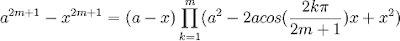Proof:

(1) Assume that n is odd.

(2) Then from Theorem 2 above, we have:(3) Since n is odd, there exists m such that n = 2m+1.

(4) This then gives us:(5) Setting x = (a/x) gives us:(6) Multiplying both sides by x2m+1 gives us:(7) Assume that n is even.

(8) Then, from Theorem 2 above we have:(9) Since n is even, there exists an integer m such that n = 2m.

(10) This then gives us:(11) Setting x = (a/x) gives us:(12) Multiplying both sides by x2m gives us:QED

Theorem 3:

If n is odd:If n is even:Proof:

(1) From Corollary 1.2 above, we have:(2) Now, it is also possible to pair up roots. That is, let l = n - k -1.

(3) Then we have:

cos([2(n-k-1)+1]π/n) =
=cos([2n - 2k -1]π/n) =
=cos([2nπ/n] - [(2k+1)π/n] ) =
=cos(2π - (2k+1)π/n) =
cos(-[2k+1]π/n) = cos([2k+1]π/n) [See Property 9, here]

sin([2(n-k-1)+1]π/n) =
=sin([2n - 2k - 1]π/n) =
=sin([2nπ/n] - [(2k+1)π/n]) =
=sin(2π - (2k+1)π/n) =
=sin(-[2k+1]π/n) = -sin([2k+1]π/n) [See Property 4, here]

(4) So, pairing each roots of unity k with its n-k-1 pair gives us: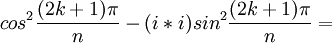(5) If n is even, the we have:(6) If n is odd, then at k=(n-1)/2: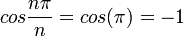and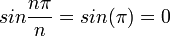(7) So:= x - (-1) - i*0 = x + 1

(8) So, if n is odd, we have:since we can see that (n-1)/2 is not included in any of the pairings:

k = 0 .. (n-1)/2 - 1

n-k-1 = (n-1)/2 + 1 .. n-1

since:

n-[(n-1)/2 - 1] - 1 =
= n - (n-1)/2 + 1 - 1 =
= (2n - [n-1])/2 =
= (n + 1)/2 =
= (n - 1 + 2)/2 =
(n-1)/2 + 1

QED

Corollary 3.1: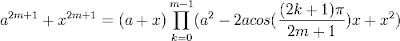Proof:

(1) Assume that n is odd.

(2) Then from Theorem 3 above, we have:(3) Since n is odd, there exists m such that n = 2m+1.

(4) This then gives us:(5) Setting x = (a/x) gives us:(6) Multiplying both sides by x2m+1 gives us:(7) Assume that n is even.

(8) Then, from Theorem 3 above we have:(9) Since n is even, there exists an integer m such that n = 2m.

(10) This then gives us:(11) Setting x = (a/x) gives us:(12) Multiplying both sides by x2m gives us:QED

References